PCA for Stock Market Analysis with scikit-learn
Mar 27, 21
Principal Components Analysis is an interesting tool that can be used for stock market analysis. Using Python, it is even possible to use it without diving too deep into the mathematics.Principal Components Analysis (PCA) is a statistical process that helps summarize data sets with a large number of variables into smaller sets of principal components. This is a powerful method for visualizing clusters and performing dimension reduction.

In this article, I will be demonstrating how PCA can be used in analyzing the stock market.

How PCA works

The PCA process can be summarized into the following 4 steps:

1. Standardize the data
2. Compute the covariance matrix
3. Find the eigenvalues and eigenvectors for the covariance matrix
4. Compute the orthogonal projection matrix and project the data onto the subspace spanned by the eigenvectors

Understandably, this may sound alien to anybody who has not taken a linear algebra class. Thankfully, the scikit-learn Python package abstracts all these away and gives us a way to perform PCA without diving into the nitty-gritty matrix computations.

Preparing the data

I used the daily returns of composite stocks of the Dow Jones Industrial Average (DJIA) for my analysis. This index was selected because there are only 30 stocks to work with, making it easier for demonstration, and still rather diverse in terms of sector representation.

As always, I imported the necessary libraries.

``````import numpy as np
import pandas as pd
import requests
import json
import time
import matplotlib.pyplot as plt
from datetime import datetime
from sklearn.preprocessing import StandardScaler
from sklearn.decomposition import PCA``````

I also created the function to retrieve financial data from the AlphaVantage API as shown in my previous article.

``````def retrieve_data(function: str, symbol: str, api_key: str) -> dict:
"""
Retrieves data from AlphaVantage's open API.
Documentation located at: https://www.alphavantage.co/documentation
"""
# query from API
url = f'https://www.alphavantage.co/query?function={function}&symbol={symbol}&apikey={api_key}'
response = requests.get(url)
data = response.text
# parse output

return parsed``````

Using the function, I queried for the SPDR Dow Jones Industrial Average ETF Trust (ticker symbol: DIA), which is an ETF that tracks the DJIA. I would need the daily close prices to compute the daily returns, so upon inspecting the data returned from the API call, I identified that the information I needed was in the "Time Series (Daily)" key.

``````index = 'DIA'
price_json = retrieve_data('TIME_SERIES_DAILY', index, api_key)``````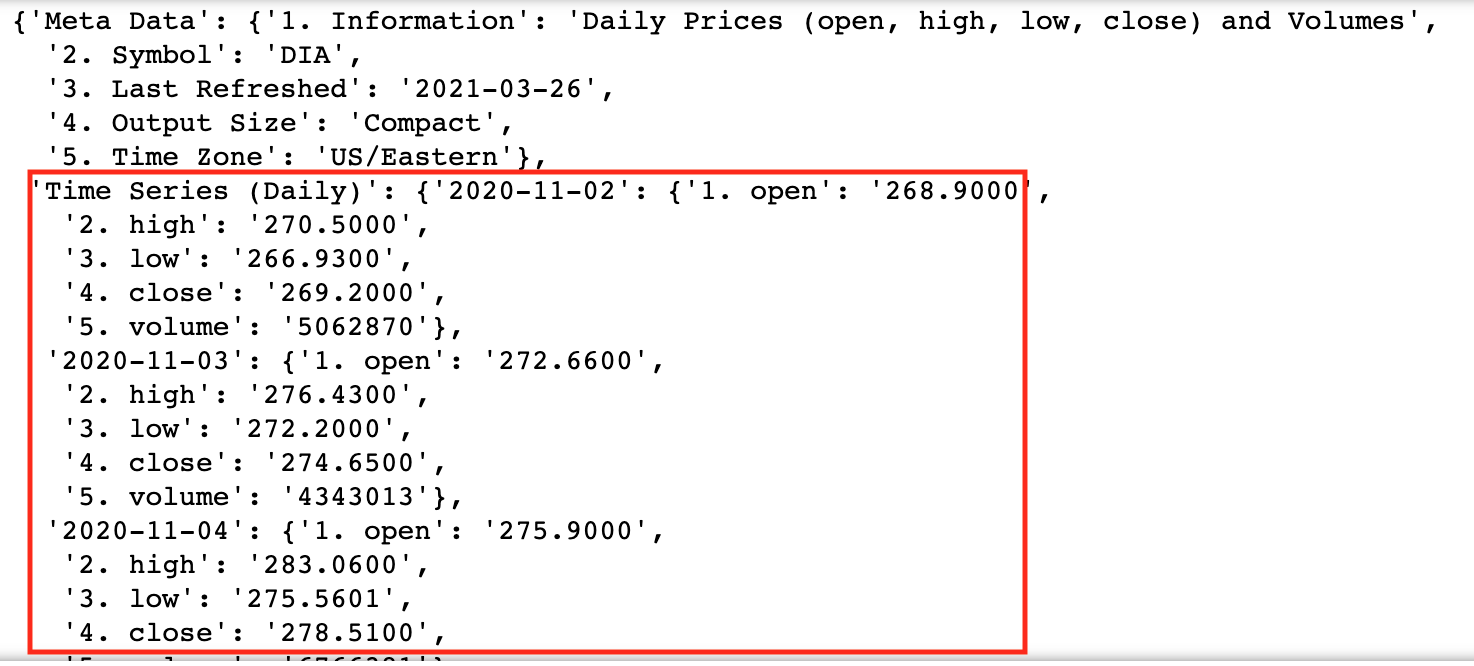I could then extract the data I needed by iterating through the list of "Time Series (Daily)" and then loading the extracted data into a Pandas data frame.

``````# extract relevant data
dates = []
close_price = []
for date, data in price_json['Time Series (Daily)'].items():
dates.append(datetime.strptime(date, '%Y-%m-%d'))
close_price.append(float(data['4. close']))

# load relevant data into data frame
df_ts = pd.DataFrame({'Date': dates, 'Close_Price': close_price})``````

Similar to my previous article on computing the Relative Strength Index, I could get the previous close price for each day by copying the close price column and shifting them up by a row. Using the day's close price and previous close price, I could then compute the daily return as (close price / previous close price) - 1 and load it into a new data frame.

``````# calculate daily return
df_ts['Previous_Close_Price'] = df_ts['Close_Price'].shift(periods=-1)
df_ts['Daily_Return'] = df_ts['Close_Price']/df_ts['Previous_Close_Price'] - 1

# load daily return into new data frame
df_daily_returns = pd.DataFrame(data=df_ts['Daily_Return'].tolist(), columns=[index])``````

Next, I had to obtain the daily returns for the DJIA composite stocks. I had created a CSV file that contained a list of the composite stocks, as well as their ticker and sector information, so I read the file into a data frame.

``DJI = pd.read_csv('DJI_composition.csv')``

I could just iterate through each stock symbol and follow similar steps as the DIA fund to obtain and prepare the daily return data for each stock.

``````DJI_symbols = DJI['Symbol'].tolist()

# iterate through each symbol
for symbol in DJI_symbols:
try:
price_json = retrieve_data('TIME_SERIES_DAILY', symbol, api_key)

# extract relevant data
dates = []
close_price = []
for date, data in price_json['Time Series (Daily)'].items():
dates.append(datetime.strptime(date, '%Y-%m-%d'))
close_price.append(float(data['4. close']))

# load relevant data into data frame
df_ts = pd.DataFrame({'Date': dates, 'Close_Price': close_price})

# calculate daily return
df_ts['Previous_Close_Price'] = df_ts['Close_Price'].shift(periods=-1)
df_ts['Daily_Return'] = df_ts['Close_Price']/df_ts['Previous_Close_Price'] - 1

df_daily_returns[symbol] = df_ts['Daily_Return'].tolist()

time.sleep(15) # to handle limited number of calls permitted in AlphaVantage's free tier
except:

Lastly, I dropped the last row in the data frame which contained NA values, and transposed the data frame. The transposing is to ensure that each row represented the returns for a stock.

``````df_daily_returns.dropna(inplace=True)
df_daily_returns_trans = df_daily_returns.transpose()``````

Finding the principal components

From the transposed data frame, I extracted only the returns of the composite stocks and standardized them using the StandardScaler function from the preprocessing package in scikit-learn.

``````x = df_daily_returns_trans.loc[DJI_symbols, :].values
x = StandardScaler().fit_transform(x)``````

With the help of the decomposition package in scikit-learn, I could simply fit the standardized data into the PCA class to obtain the principal components. For this case, I would only need to get 2 principal components but it is possible to specify more or less.

``````pca = PCA(n_components=2)
components = pca.fit_transform(x)``````

The principal components were then loaded into a data frame and the output looks something like this:

``````df_components = pd.DataFrame(
data=components,
columns=['pc1', 'pc2']
)``````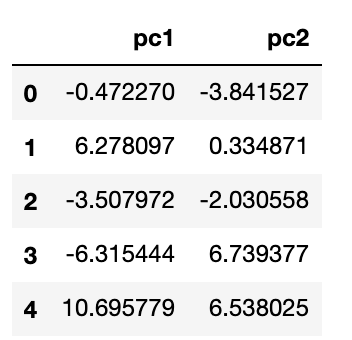Visualizing clustering

We could do a quick visualization by plotting the principal components against each other in a scatter plot.

``df_components.plot.scatter(x='pc1', y='pc2')``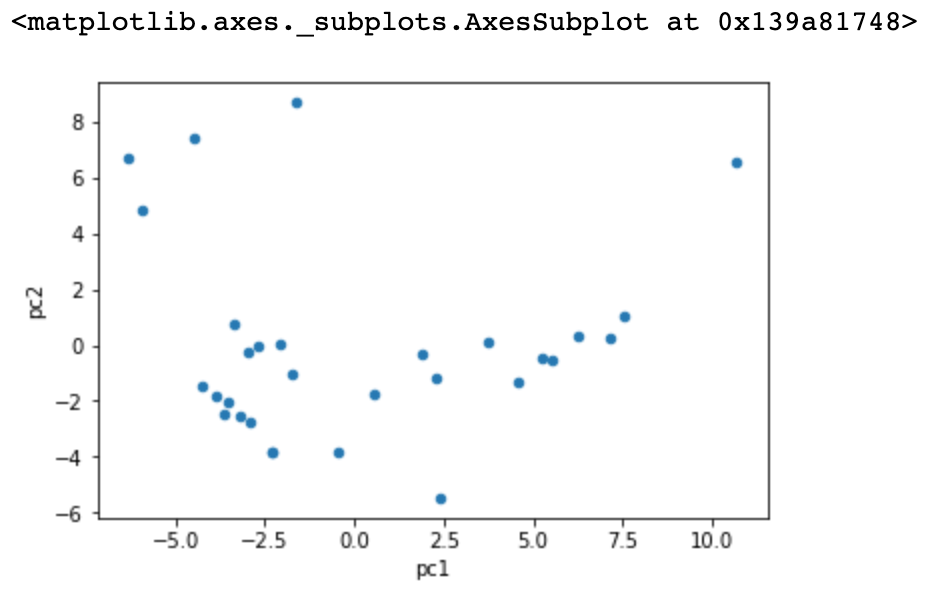Just from this plot alone, we can see some clustering, suggesting that these companies are similar to each other. To verify this, we can add the sector information to each stock and replot the principal components.

``````# add sector information
df_components['sector'] = DJI['Sector']\

# get unique sectors
sectors = list(set(DJI['Sector'].tolist()))

# plot
fig=plt.figure(figsize = (8,8))
ax.set_xlabel('Principal Component 1', fontsize = 12)
ax.set_ylabel('Principal Component 2', fontsize = 12)
cmap = plt.cm.get_cmap('hsv', len(sectors))
for i in range(len(sectors)):
ax.scatter(
x=df_components[df_components['sector'] == sectors[i]]['pc1'],
y=df_components[df_components['sector'] == sectors[i]]['pc2'],
c=cmap(i),
s=50
)
ax.legend(sectors)``````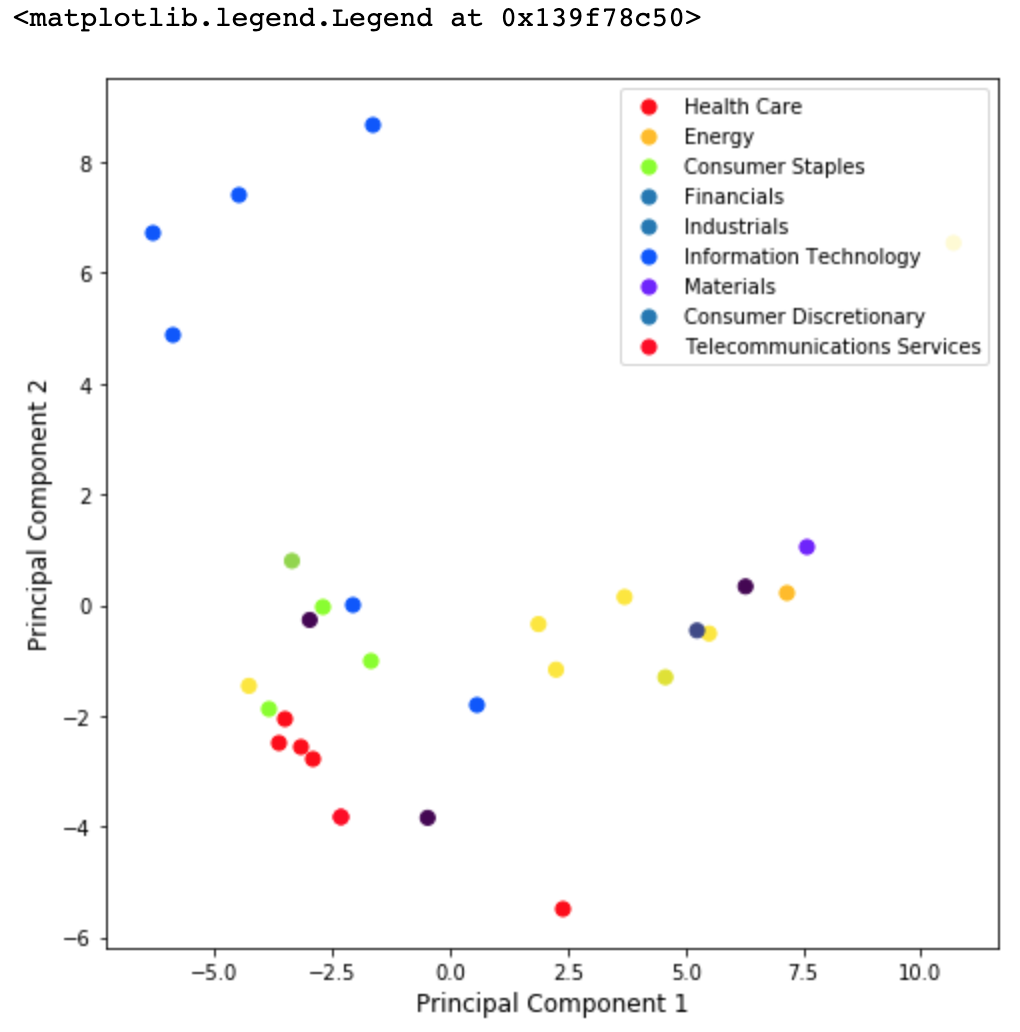We can see that just among these 30 stocks, stocks of the same sector tend to cluster together. This would be even clearer if more stocks were added to the analysis.

Mimicking index returns

I also wanted to see if the principal components generated would be useful in helping me replicate the DJIA returns. To test my hypothesis, I used the first principal component, which has the best explanatory power for the variance in the data and turned the principal component for each stock into a weight. I then fetched the transposed daily returns for the composite stocks and adjusted them by their corresponding weights.

``````weights = abs(df_components['pc1'])/sum(abs(df_components['pc1']))
df_plot = pd.DataFrame(df_daily_returns_trans.loc[DJI_symbols, :])
df_plot['weights'] = weights.tolist()

# iterate through each column except weights
for i in range(df_plot.shape - 1):
df_plot.iloc[:, i] = df_plot.iloc[:, i] * df_plot['weights']

# drop weights column
df_plot = df_plot.drop(['weights'], axis = 1)``````

I plotted them to see how my portfolio would have performed with the calculated weights.

``df_plot.transpose().sum(1).plot()``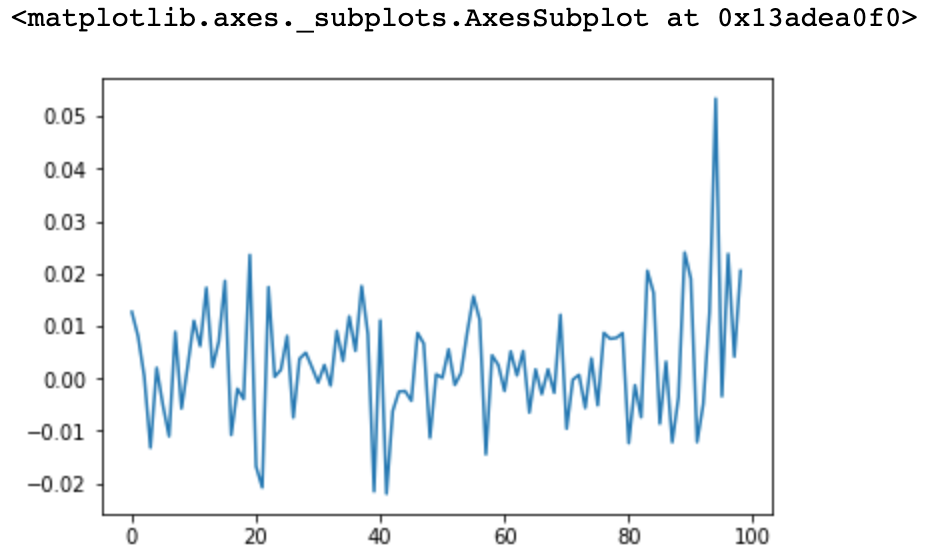I then plotted the actual DIA returns for comparison.

``df_daily_returns[index].plot()``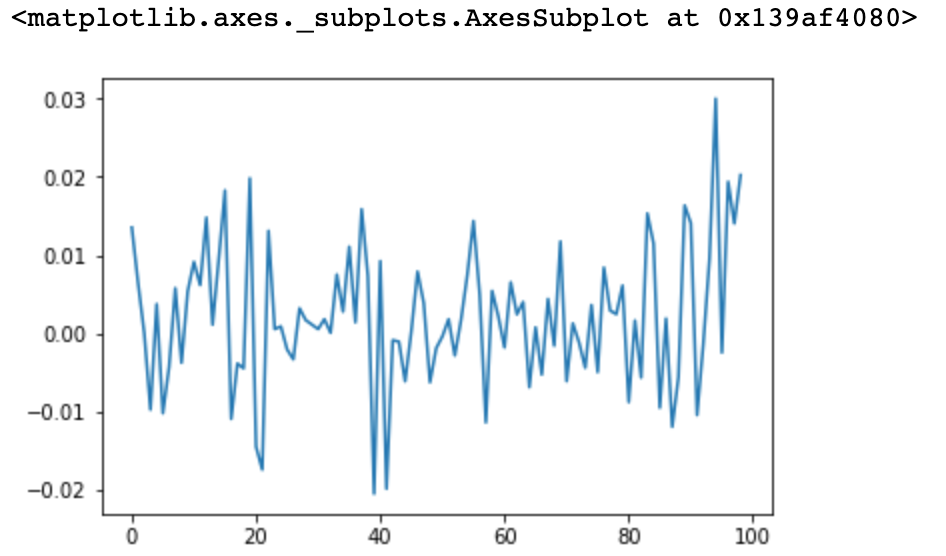Notice how similar the plots are to each other. This means that if I had maintained a portfolio with the stock weights based on PCA, it would have had pretty similar returns to the DIA ETF, and thus the DJIA index.